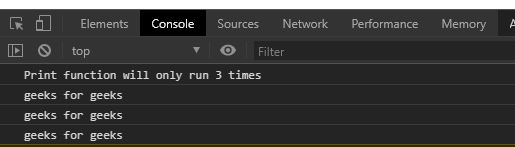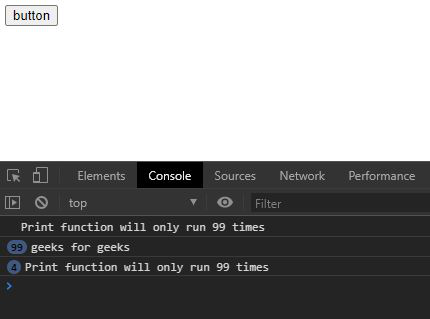# Underscore.js _.before() function

_.before() function in underscore.js is used to call a particular function a particular number of times. It uses a count variable that keeps the track of number of times the function is called.

Syntax:

`_.before(count, function)`

Parameters:

• Count: It is the number of times a function is to be called.
• Function: It is the function that runs the count times.

Return: This function returns the the count of the function call.

Note: Please Link the underscore CDN before using this code directly in the browser through the code.

Example 1:

 `  ` `<``html``>  ` `  ``<``head``>  ` `    ``<``script` `src` `=   ` `"https://cdnjs.cloudflare.com/ajax/libs/underscore.js/1.9.1/underscore-min.js"` `>  ` `    ``  ` `  ``  ` `  ``<``body``>  ` `    ``<``script``> ` `      ``let print=()=>{ ` `        ``console.log("geeks for geeks") ` `      ``} ` `      ``console.log(`Print function will only run 3 times`) ` `      ``//the print function cannot run more than 3 times ` `      ``count=_.before(4, print); ` `      ``//calling count function more than 3 times ` `      ``count(); ` `      ``count(); ` `      ``count(); ` `      ``count(); ` `      ``count(); ` `      ``count(); ` `      ``count(); ` `    `` ` `  ``  ` ``

Output:Example 2:

It is clear from the example that if The button is clicked again and again than print function will not be working as it as already reached the count.

 `  ` `<``html``>  ` `  ``<``head``>  ` `    ``<``script` `src` `=   ` `"https://cdnjs.cloudflare.com/ajax/libs/underscore.js/1.9.1/underscore-min.js"` `>  ` `    ``  ` `   ``  ` `  ``<``body``>  ` `    ``<``button` `id``=``"btn"``>button ` `    ``<``script``> ` `      ``let print=()=>{ ` `        ``console.log("geeks for geeks") ` `      ``} ` `      ``//the print function cannot run more than 99 times ` `      ``count=_.before(100, print); ` `      ``//calling count function more than 99 times ` `      ``let btn=document.querySelector("#btn"); ` `      ``let run=()=>{ ` `      ``console.log(`Print function will only run 99 times`) ` `        ``for(let i=0; i<``5000``; i++){ ` `          ``count() ` `        ``} ` `      ``} ` `      ``btn.addEventListener("click", run) ` `    `` ` `  ``  ` ``

Output:My Personal Notes arrow_drop_upCheck out this Author's contributed articles.

If you like GeeksforGeeks and would like to contribute, you can also write an article using contribute.geeksforgeeks.org or mail your article to contribute@geeksforgeeks.org. See your article appearing on the GeeksforGeeks main page and help other Geeks.

Please Improve this article if you find anything incorrect by clicking on the "Improve Article" button below.